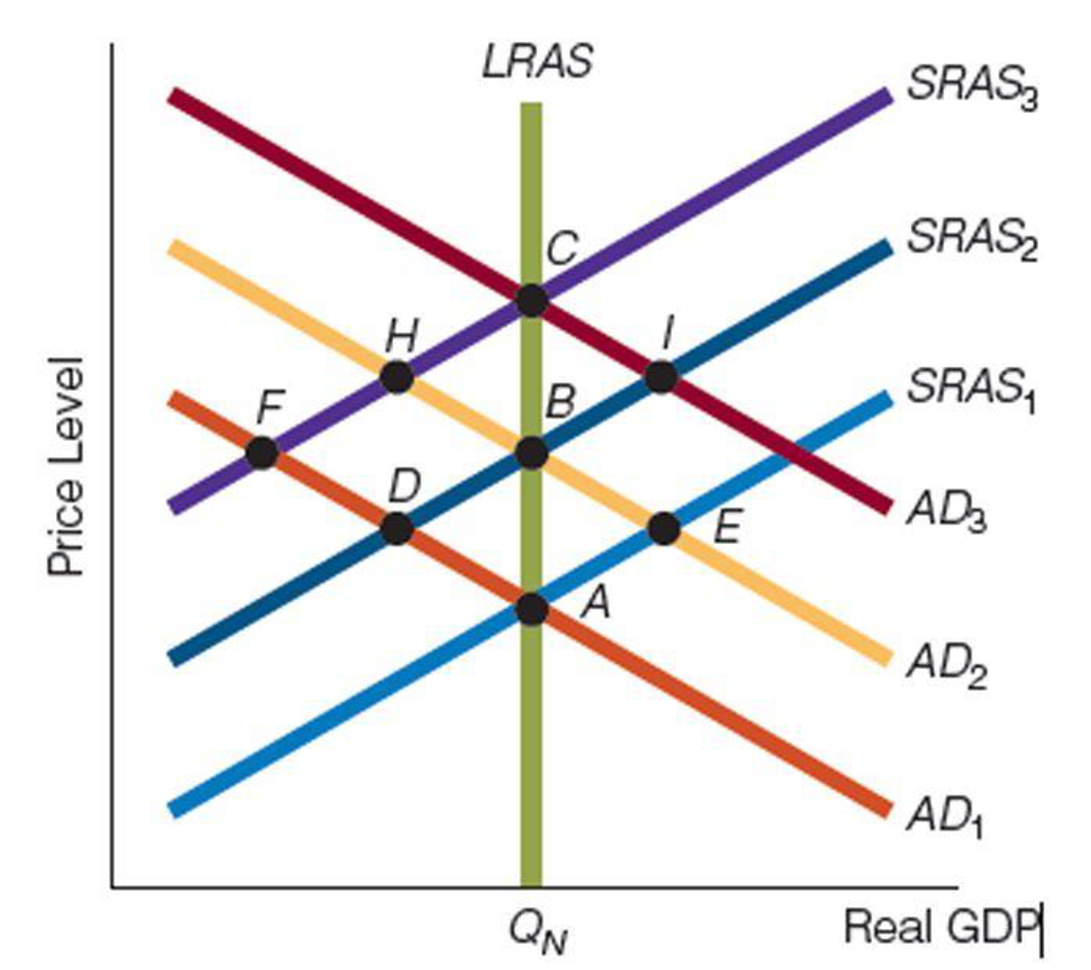Chapter 14, Problem 3WNG

Chapter
Section
Textbook Problem

Use the accompanying figure to answer questions a and b.a. Suppose the economy is self-regulating and is at point A when there is a one-shot, demand-induced inflation. If there are no other changes in the economy, at what point will the economy settle? b. Suppose the economy is at point A when it is faced with two adverse supply shocks. The Fed tries to counter these shocks by increasing aggregate demand. What path will the economy follow?

(a)

To determine

Effect of demand induced inflation in self-regulating economy.

Explanation

Suppose the economy is self-regulating an increase in the demand will lead to increase the price of the good and it supply will shown in the below diagram.

According to the diagram, initially the economy is at the point 1, were the economy producing sufficient output. Assuming that there is an increase in demand, the demand curve AD1 shift to AD2 and the economy move to point 2...

(b)

To determine

Path of the economy when the adverse supply shock is corrected with increasing aggregate demand.

Still sussing out bartleby?

Check out a sample textbook solution.

See a sample solution

The Solution to Your Study Problems

Bartleby provides explanations to thousands of textbook problems written by our experts, many with advanced degrees!

Get Started

Find more solutions based on key concepts